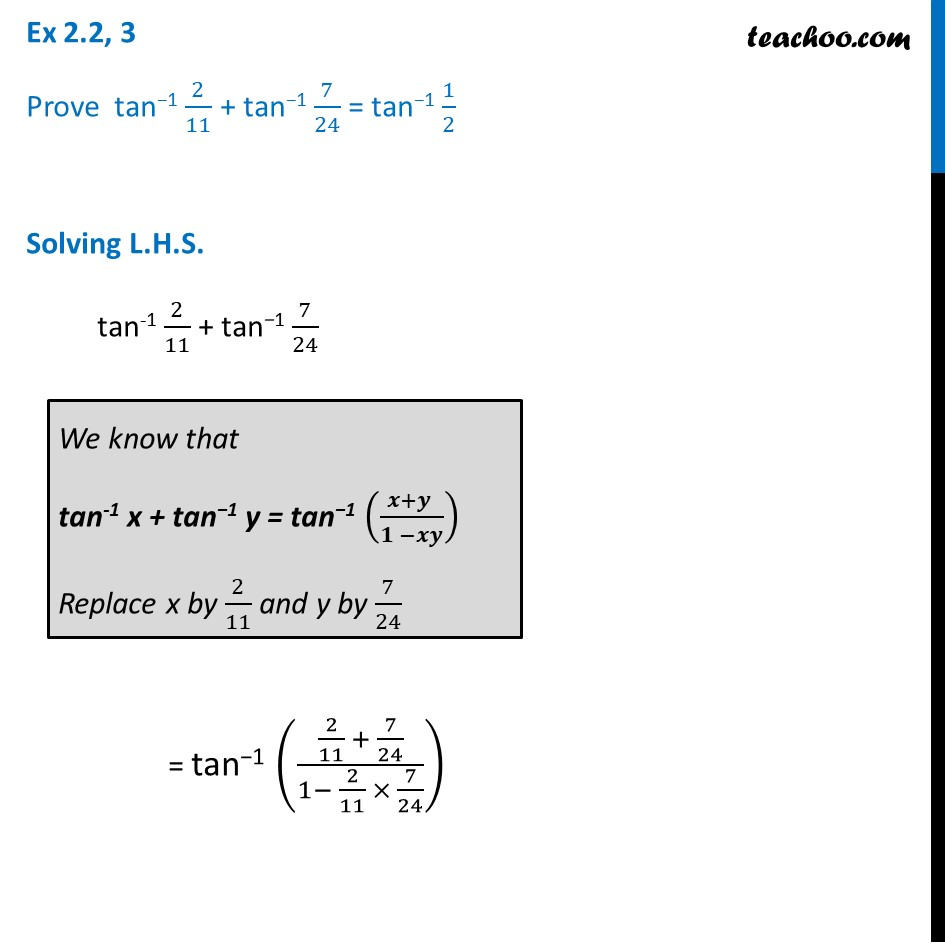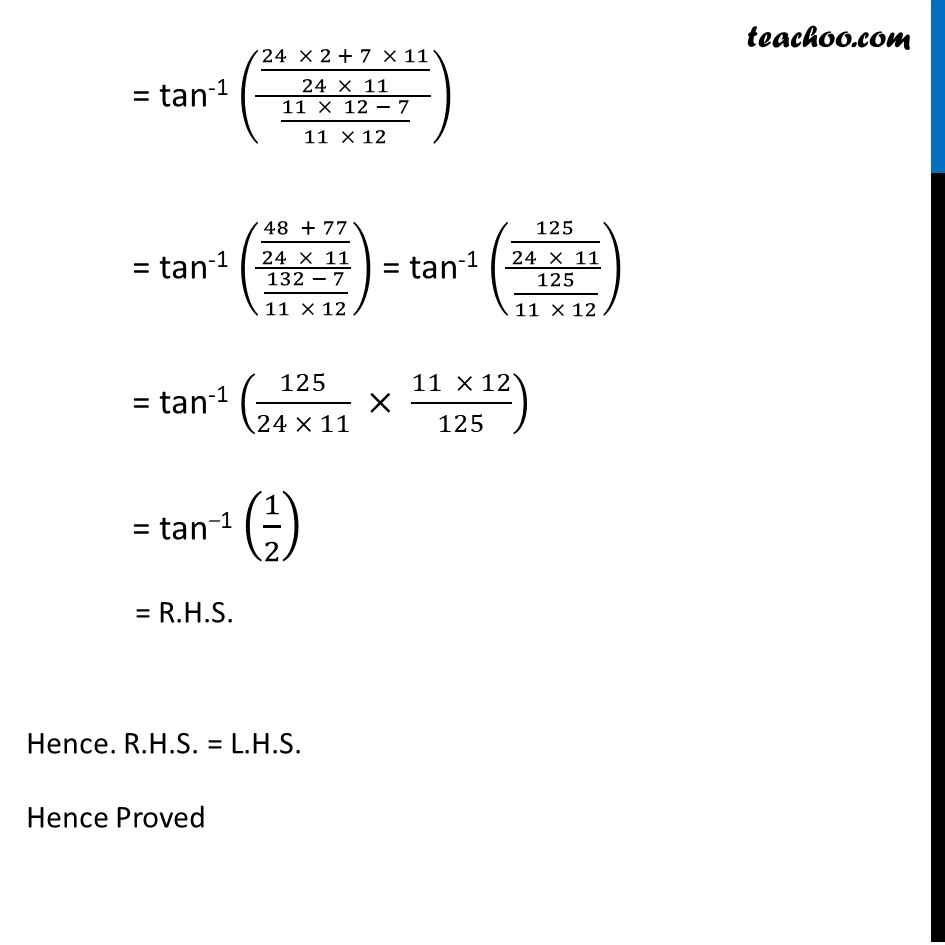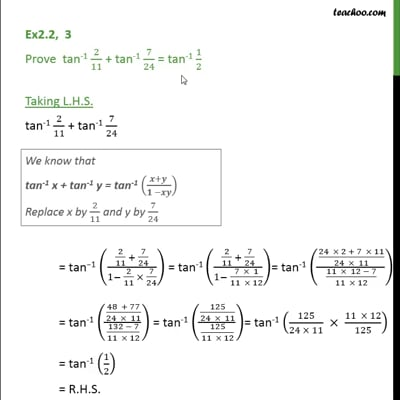Ex 2.2

Chapter 2 Class 12 Inverse Trigonometric Functions
Serial order wiseThis video is only available for Teachoo black users

Solve all your doubts with Teachoo Black (new monthly pack available now!)

### Transcript

Ex 2.2, 3 Prove tan−1 2/11 + tan−1 7/24 = tan−1 1/2 Solving L.H.S. tan-1 2/11 + tan−1 7/24 = "tan−1" ((2/11 + 7/24)/(1− 2/11 × 7/24)) We know that tan-1 x + tan−1 y = tan−1 ((𝒙+𝒚 )/(𝟏 −𝒙𝒚)) Replace x by 2/11 and y by 7/24= tan-1 (((24 × 2 + 7 × 11)/(24 × 11))/((11 × 12 − 7)/(11 × 12))) = tan-1 (((48 + 77)/(24 × 11))/((132 − 7)/(11 × 12))) = tan-1 ((125/(24 × 11))/(125/(11 × 12))) = tan-1 (125/(24 × 11) × (11 × 12)/125) = tan−1 (1/2) = R.H.S. Hence. R.H.S. = L.H.S. Hence Proved